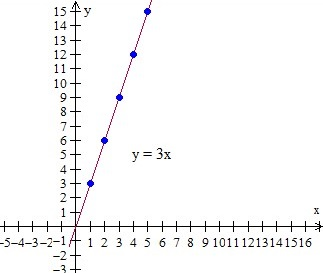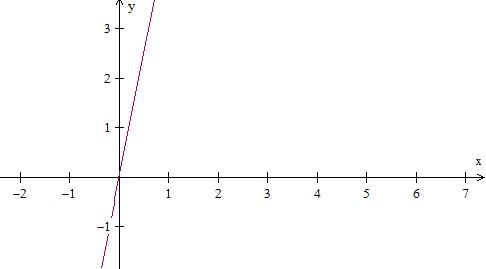# Making a table and plotting points given a unit rate

#### Excel Pivot Tables and Dashboard. Simple & Quick tutorial!

Most Popular

7 Lectures 58 mins

#### Learn HTML Tables

15 Lectures 59 mins

#### Excel Analytics - Data Analysis with Pivot-Tables and Charts

46 Lectures 2.5 hours

Unit rate is defined as a ratio in which denominator is 1. In general, a unit rate is the number of dependent variables per one independent variable.

For example − A monkey eats 8 bananas in 2 minutes

The unit rate here is the number of bananas the monkey eats in a minute.

Unit rate = 8/2 = 4 bananas per minute.

Unit rate and table

If we have a proportional relationship like for example $9 for 3 kg, we can make a table of values and graph the relationship. We already have learnt about unit rate. In this case the unit rate is the number of dollars per 1 kg. Here the unit rate is found as$9/3kg = $3/kg. In a table or graph, representing proportional relationships, the constant of proportionality and the unit rate are same. Given a unit rate we can write pairs of x and y values following the proportional relationship as follows. For example if the unit rate is$3/kg we can write (1kg, $3), (2 kg,$6), (3 kg, $9), (4 kg,$12), (5 kg, $15) and so on. We then put these ordered pairs in a table as follows. Weight in kg(x) Cost in$(y)
1 3
2 6
3 9
4 12
5 15

Unit rate and graph

The proportional relationship for given rate \$3/kg can be written as an equation y = 3x. The same can be plotted as a graph given below.Given a unit rate, we can write a function rule like y = mx, where m is the unit rate, x and y are the variables. Using this rule we can find points with x and y values which can be put in a table. Then we plot these points on a graph.

The relationship having unit rate is given by the equation: y = 2x + 1 Make a table and plot 5 points.

### Solution

Step 1:

In the equation y = 2x + 1,

For x = 0, y = 1, for x = 1, y = 3 and so on

For different values of x, we get the following table

Input(x) Output(y)
0 1
1 3
2 5
3 7
4 9

Step 2:

Plotting these points we get this graphThe relationship having unit rate is given by the equation: y = 5x. Make a table and plot 5 points.

### Solution

Step 1:

In the equation y = 5x

For x = 0, y = 0, for x = 1, y = 5 and so on

For different values of x, we get the following table

Input(x) Output(y)
0 0
1 5
2 10
3 15
4 20

Step 2:

Plotting these points we get this graph